# 使用 SUMO 进行仿真(2)-Node 和 Edge2021年9月24日07:43:00

8261字阅读27分32秒

## 简介

• `Network` 的创建，关于 `node``edge` 的用法；
• Edge Type 的使用，设置 edge 的属性，来简化 edge 文件；
• 利用 Open Street Map 来生成路网；

### 参考资料

• 知乎上关于 sumo 的一个教程文章, 还是很不错的：
• 本文的相关代码参考 Github 仓库，SUMO Tutorial`Node_Edge_Introduce` 文件夹部分）

## Network 文件生成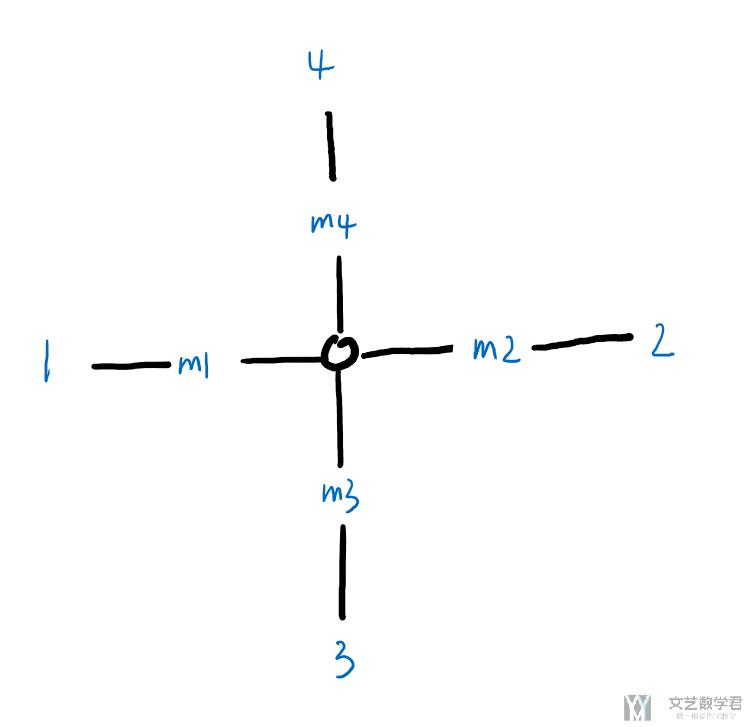### Node 的相关说明 (Node Descriptions)

1. <nodes> <!-- The opening tag -->
2.   <node id="0" x="0.0" y="0.0" type="traffic_light"/> <!-- def. of node "0" -->
3.   <node id="1" x="-500.0" y="0.0" type="priority"/> <!-- def. of node "1" -->
4.   <node id="2" x="+500.0" y="0.0" type="priority"/> <!-- def. of node "2" -->
5.   <node id="3" x="0.0" y="-500.0" type="priority"/> <!-- def. of node "3" -->
6.   <node id="4" x="0.0" y="+500.0" type="priority"/> <!-- def. of node "4" -->
7.   <node id="m1" x="-250.0" y="0.0" type="priority"/> <!-- def. of node "m1" -->
8.   <node id="m2" x="+250.0" y="0.0" type="priority"/> <!-- def. of node "m2" -->
9.   <node id="m3" x="0.0" y="-250.0" type="priority"/> <!-- def. of node "m3" -->
10.   <node id="m4" x="0.0" y="+250.0" type="priority"/> <!-- def. of node "m4" -->
11. </nodes> <!-- The closing tag -->### Edge 的相关说明 (Edge Descriptions)

• id, 每一个 edge 独特的标志, 用来找到指定的 edge；
• from, to，from 和 to 是与上面的 node 的 id 对应的)
• priority，优先级, 在转弯处有用；
• numLanes，这个edge上包含多少个车道；
• speed，车道的车速，也就是限速；

1. <edges>
2.   <edge id="1fi" from="1" to="m1" priority="2" numLanes="2" speed="11.11"/>
3.   <edge id="1si" from="m1" to="0" priority="3" numLanes="3" speed="13.89"/>
4.   <edge id="1o" from="0" to="1" priority="1" numLanes="1" speed="11.11"/>
5.   <edge id="2fi" from="2" to="m2" priority="2" numLanes="2" speed="11.11"/>
6.   <edge id="2si" from="m2" to="0" priority="3" numLanes="3" speed="13.89"/>
7.   <edge id="2o" from="0" to="2" priority="1" numLanes="1" speed="11.11"/>
8.   <edge id="3fi" from="3" to="m3" priority="2" numLanes="2" speed="11.11"/>
9.   <edge id="3si" from="m3" to="0" priority="3" numLanes="3" speed="13.89"/>
10.   <edge id="3o" from="0" to="3" priority="1" numLanes="1" speed="11.11"/>
11.   <edge id="4fi" from="4" to="m4" priority="2" numLanes="2" speed="11.11"/>
12.   <edge id="4si" from="m4" to="0" priority="3" numLanes="3" speed="13.89"/>
13.   <edge id="4o" from="0" to="4" priority="1" numLanes="1" speed="11.11"/>
14. </edges>

• `node 4``node m4` 方向, 有 2 个车道
• `node m4``node 0` 方向, 有 3 个车道
• `node 0``node 4` 方向, 有 1 个车道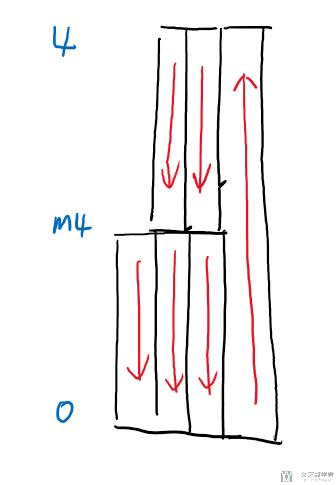1. <edge id="4fi" from="4" to="m4" priority="2" numLanes="2" speed="11.11"/> <!-- 4->m4, 2 车道 -->
2. <edge id="4si" from="m4" to="0" priority="3" numLanes="3" speed="13.89"/> <!-- m4->0, 3 车道 -->
3. <edge id="4o" from="0" to="4" priority="1" numLanes="1" speed="11.11"/> <!-- 0->4, 1 车道 -->

`netconvert --node-files=hello.nod.xml --edge-files=hello.edg.xml --output-file=hello.net.xml`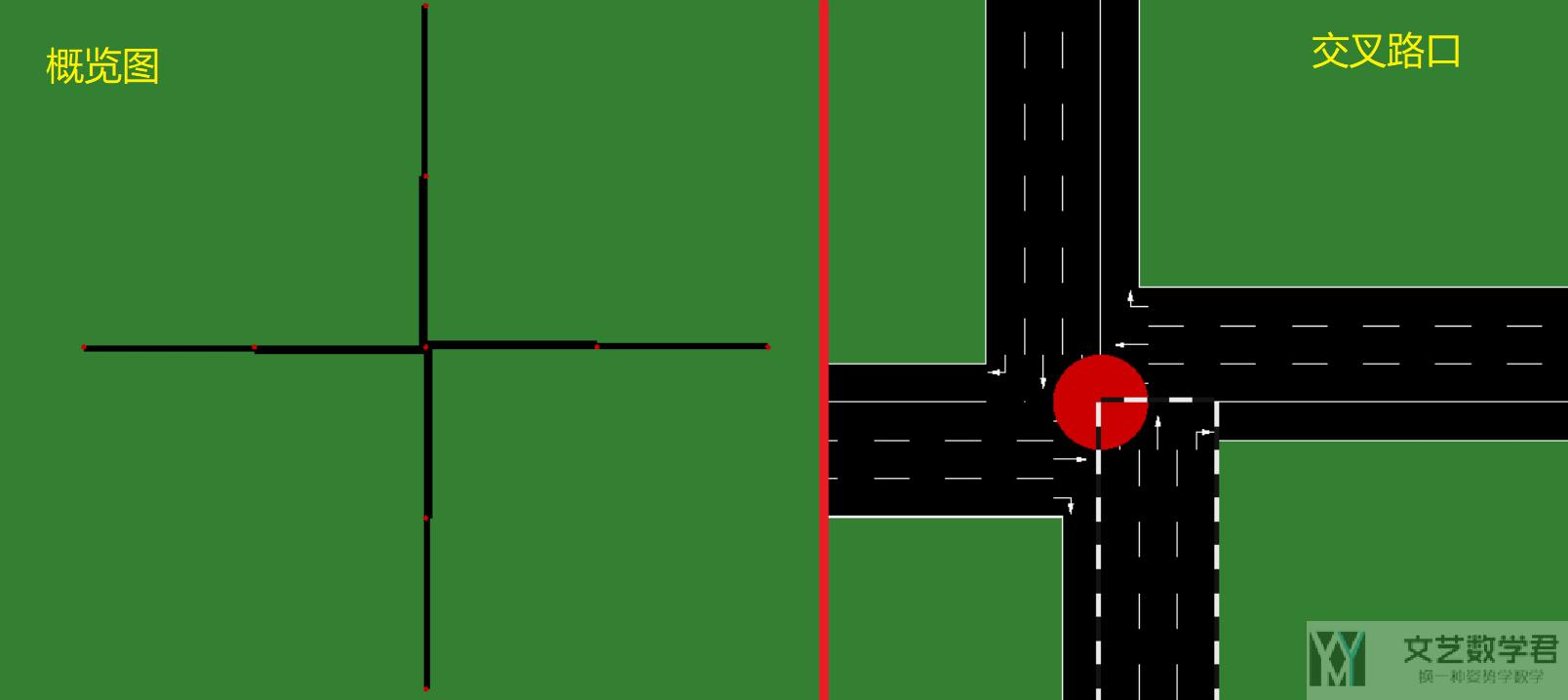#### Edge Type

1. <types>
2.   <type id="a" priority="3" numLanes="3" speed="13.889"/>
3.   <type id="b" priority="2" numLanes="2" speed="11.111"/>
4.   <type id="c" priority="1" numLanes="1" speed="11.111"/>
5. </types>

1. <edges>
2.   <edge id="1fi" from="1" to="m1" type="b"/>
3.   <edge id="1si" from="m1" to="0" type="a"/>
4.   <edge id="1o" from="0" to="1" type="c"/>
5.   <edge id="2fi" from="2" to="m2" type="b"/>
6.   <edge id="2si" from="m2" to="0" type="a"/>
7.   <edge id="2o" from="0" to="2" type="c"/>
8.   <edge id="3fi" from="3" to="m3" type="b"/>
9.   <edge id="3si" from="m3" to="0" type="a"/>
10.   <edge id="3o" from="0" to="3" type="c"/>
11.   <edge id="4fi" from="4" to="m4" type="b"/>
12.   <edge id="4si" from="m4" to="0" type="a"/>
13.   <edge id="4o" from="0" to="4" type="c"/>
14. </edges>

1. netconvert --node-files=cross3l.nod.xml --edge-files=cross3l.edg.xml --type-files=cross3l.typ.xml --output-file=cross3l.net.xml

1. ... previous definitions ...
2.   <edge id="1si" from="m1" to="0" type="a">
3.     <lane index="1" disallow="all"/>
4.   </edge>
5.   <edge id="1o" from="0" to="1" type="c"/>
6. ... further definitions ...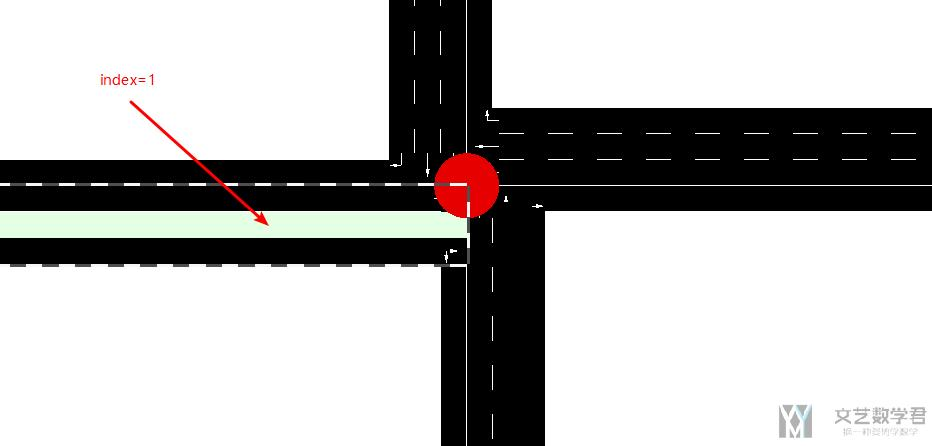1. ... previous definitions ...
2.   <edge id="2si" from="m2" to="0" priority="3" numLanes="3" speed="13.89">
3.     <split pos="0" lanes="0 1"/>
4.     <split pos="30" lanes="0 1 2" speed="10"/>
5.   </edge>
6. ... further definitions ...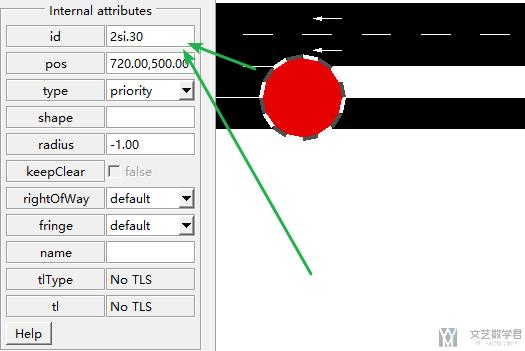node 与 node 之间只包含参数 lanes 中的 lane。例如从起点 0m 到 30m 的这一段，就只包含 2 个 lane。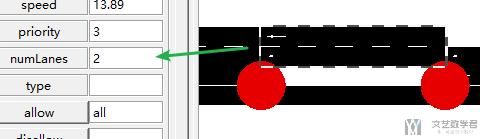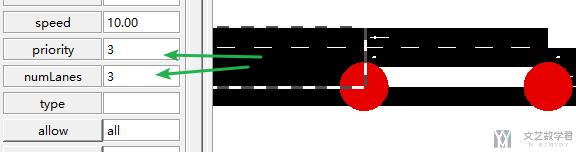## 生成网格状地图

1. # 生成Node文件, 从左下角(0,0)开始生成的
2. with open('cross.nod.xml', 'w') as file:
3.     file.write('<nodes> \n')
4.     for i in range(6):
5.         for j in range(6):
6.             file.write('<node id="node%d" x="%d" y="%d" type="priority" /> \n' %(i*6+j, i*100, j*100))
7.     file.write('</nodes> \n')

1. # 生成edge文件
2. with open('cross.edg.xml', 'w') as file:
3.     file.write('<edges> \n')
4.     for i in range(6):
5.         for j in range(6):
6.             k=0
7.             if i > 0:
8.                 file.write('<edge id="edge%d_%d" from="node%d" to="node%d" priority="75" numLanes="2" speed="40" /> \n' % (i*6+j,k,i*6+j,(i-1)*6+j))
9.                 k=k+1
10.             if i < 5:
11.                 file.write('<edge id="edge%d_%d" from="node%d" to="node%d" priority="75" numLanes="2" speed="40" /> \n' % (i*6+j,k,i*6+j,(i+1)*6+j))
12.                 k=k+1
13.             if j > 0:
14.                 file.write('<edge id="edge%d_%d" from="node%d" to="node%d" priority="75" numLanes="2" speed="40" /> \n' % (i*6+j,k,i*6+j,i*6+j-1))
15.                 k=k+1
16.             if j < 5:
17.                 file.write('<edge id="edge%d_%d" from="node%d" to="node%d" priority="75" numLanes="2" speed="40" /> \n' % (i*6+j,k,i*6+j,i*6+j+1))
18.                 k=k+1
19.     file.write('</edges> \n')

1. netconvert --node-files=cross.nod.xml --edge-files=cross.edg.xml --output-file=cross.net.xml### Network 文件说明

• “s” = straight
• “t” = turn
• “l” = left
• “r” = right
• “L” = partially left
• “R” = partially right
• “invalid” = no direction

## 从 Open Street Map 生成路网

### 获取路网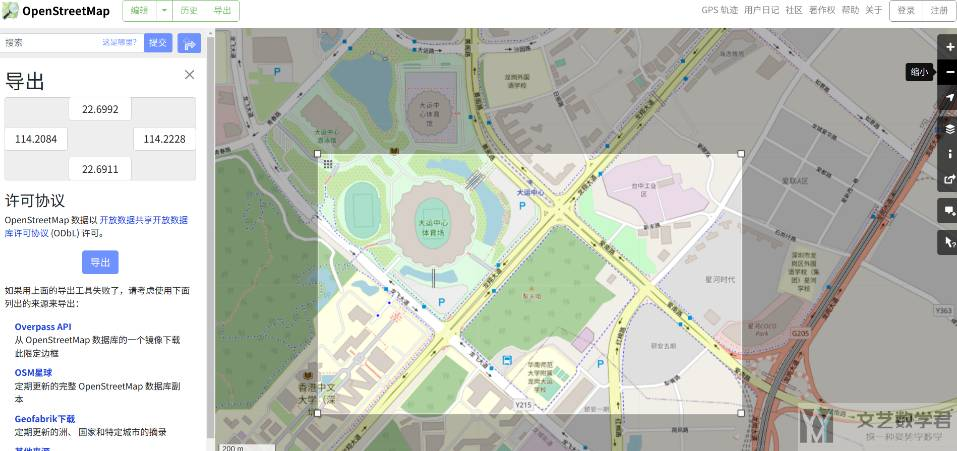### 路网转换

1. netconvert --osm-files map.osm  --geometry.remove --ramps.guess --junctions.join --tls.guess-signals --tls.discard-simple --tls.join --tls.default-type actuated -o sumo.net.xml

• --geometry.remove : Simplifies the network (saving space) without changing topology
• --ramps.guess : Acceleration/Deceleration lanes are often not included in OSM data. This option identifies roads that likely have these additional lanes and adds them
• --junctions.join : See #Junctions，这个可以将附近的交叉路口进行合并；
• --tls.guess-signals --tls.discard-simple --tls.join : See #Traffic_Lights
• --tls.default-type actuated : Default static traffic lights are defined without knowledge about traffic patterns and may work poorly in high traffic

• 删除多余的道路，例如人行道，地铁等；
• 修改车道数，可以根据卫星图片进行校对；

• 微信公众号
• 关注微信公众号
•• QQ群
• 我们的QQ群号
•• 本文由 发表于 2021年9月24日07:43:00
• 转载请务必保留本文链接：https://mathpretty.com/12797.html